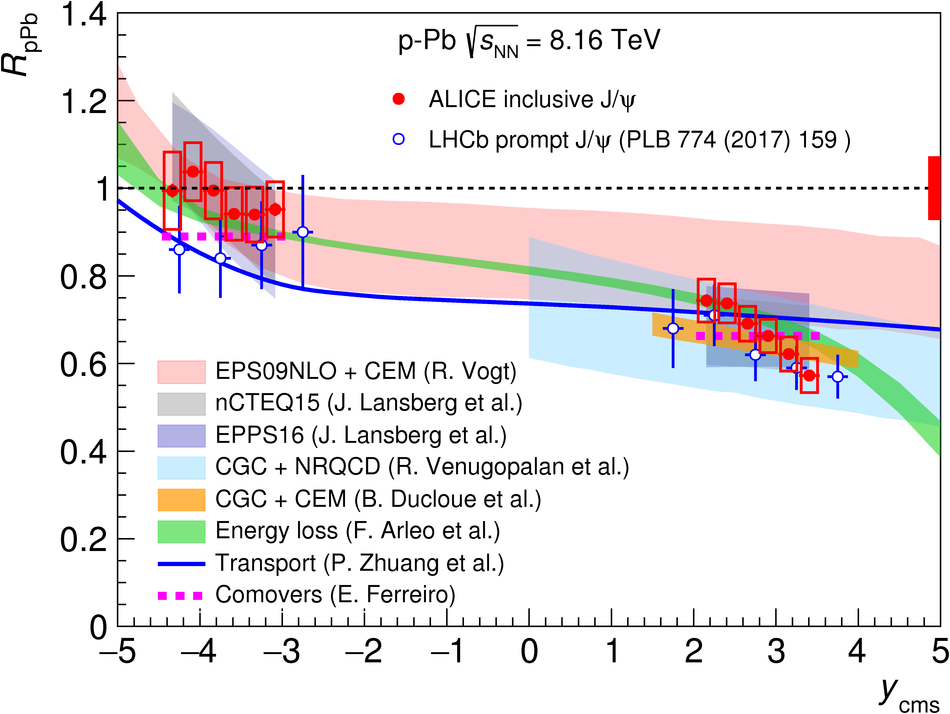# Figure 6

 Comparison of the ALICE and LHCb~ results on the $y_{\rm cms}$-dependence of the J/$\psi$ nuclear modification factors in \mbox{p--Pb} and \mbox{Pb--p} collisions at $\sqrt{s_{\rm NN}}=8.16$ TeV. The horizontal bars correspond to the bin size. For ALICE, the vertical error bars represent the statistical uncertainties, the boxes around the points the uncorrelated systematic uncertainties, and the filled box around unity the correlated uncertainties. For LHCb, the vertical error bars represent the combination of statistical and systematic uncertainties. The results are also compared to several model calculations~ (see text for details).